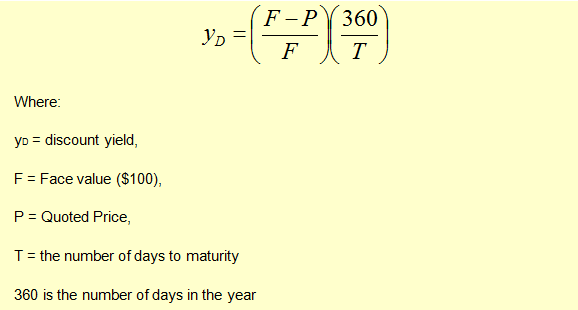# Bank Discount Yield

T-bills are quoted on a bank discount yield basis. The bank discount yield is calculated using the following formula:Let’s take an example. The quoted price for a 90-day T-bill is USD 975,342 with a face value of USD 1 million.

The bank discount yield will be calculated as follows:

yD =(1,000,000 – 975,342)/1,000,000 * 360/90 = 9.86%

The quoted yield on a bank discount basis is not useful for the following reasons:

• It is based on face value rather than actual dollar amount invested.
• It is annualized according to a 360-day rather than a 365-day year. This makes it difficult to compare T-bills with Treasury notes and bonds which pay interest on a 365 day basis.
• While annualizing the yield, it ignores the compounding effect.